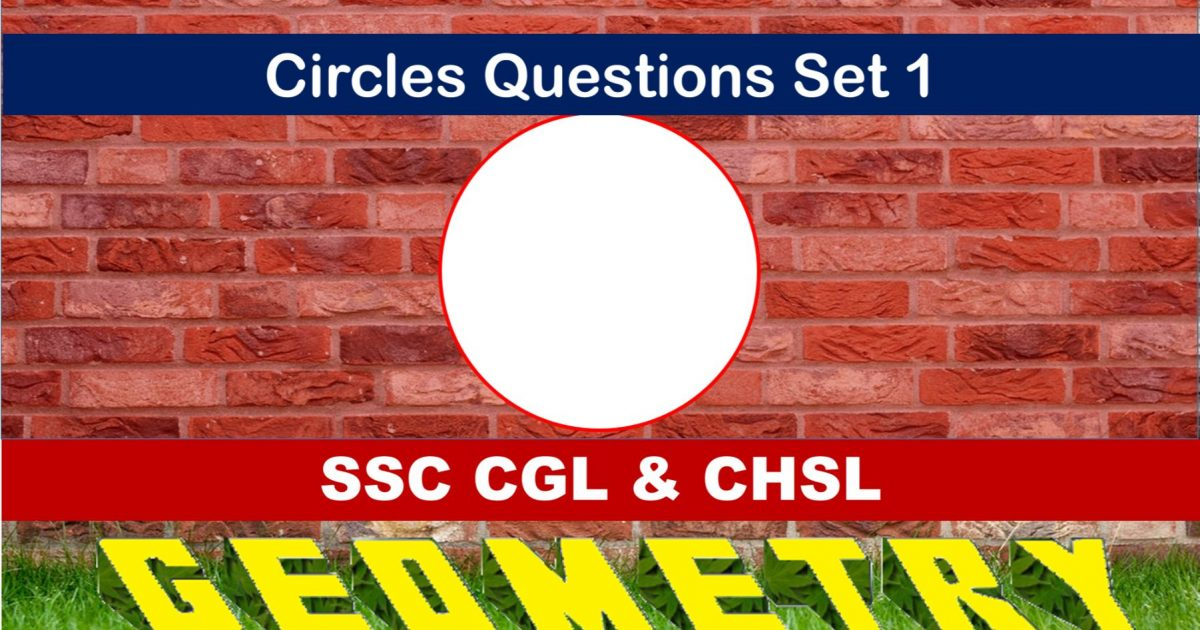Monday, September 27, 2021
Home > geometry > SSC CGL Geometry Circle Set 1

# SSC CGL Geometry Circle Set 1SSC CGL CHSL Geometry
Tangent to Circle Questions Sets
Set 1Set 2Set 3Set 4

SSC CGL Geometry Circle Set 1 : Ques No 1

In a cyclic quadrilateral ABCD, side AB is extended to E so that BE = BC. If ∠ADC = 70 degrees and ∠BAD = 95 degrees, then find ∠DCE

Options:

A. 140 degrees

B. 165 degrees

C. 120 degrees

D. 110 degrees

Solution:

SSC CGL Geometry Circle Set 1 : Ques No 2

A, B and C are three points on the circumference of a circle. If AB = AC = 5√2 cm and ∠BAC = 90 degrees, then find the length of the radius

Options:

A. 15 cm

B. 5 cm

C. 10 cm

D. 20 cm

Solution:

SSC CGL Geometry Circle Set 1 : Ques No 3

If PN is the perpendicular from a point P on the circumference of a circle of radius 7 cm to its diameter AB and the length of the chord PB is 12 cm, the length of BN is

Options:

A. 6 5/7 cm

B. 3 5/7 cm

C. 12 2/7 cm

D. 10 2/7 cm

Solution:

SSC CGL Geometry Circle Set 1 : Ques No 4

If the angle subtended by a chord at its center is 60 degrees, the ratio between the lengths of the chord and the radius is

Options:

A. 1 : 1

B. 2 : 1

C. √2 : 1

D. 1 : 2

Solution:

SSC CGL Geometry Circle Set 1 : Ques No 5

AB and CD are two parallel chords of respective lengths 8 cm and 6 cm on the same side of the center of a circle. The distance between them is 1 cm. Then, the radius of the circle is

Options:

A. 4 cm

B. 5 cm

C. 3 cm

D. 2 cm

Solution:

SSC CGL Geometry Circle Set 1 : Ques No 6

In an isosceles triangle ABC, AB = AC. A circle passing through B and touching AC at its middle point, intersects AB at P. Then AP : AB is

Options:

A. 2 : 3

B. 4 : 1

C. 1 : 4

D. 3 : 5

Solution:SSC CGL Geometry Circle Set 1 : Ques No 7

Two circles with centers at P and Q intersect each other at B and C. Two points on both sides of C are placed such on the circles that A, C, D are collinear with A on the first circle. If ∠APB = 130 degrees, then find ∠BQD

Options:

A. 130 degrees

B. 165 degrees

C. 65 degrees

D. 15 degrees

Solution:

SSC CGL Geometry Circle Set 1 : Ques No 8

The radius of the circumcircle of a right angled triangle is 15 cm and the radius of the inscribed circle is 6 cm. Then, the length of three sides (in cm) are

Options:

A. 30, 24, 25

B. 24, 36, 20

C. 18, 24, 30

D. 30, 40, 41

Solution:

SSC CGL Geometry Circle Set 1 : Ques No 9

The distance between two parallel chords of length 8 cm each in a circle of diameter 10 cm is

Options:

A. 7 cm

B. 6 cm

C. 5.5 cm

D. 8 cm

Solution:

SSC CGL Geometry Circle Set 1 : Ques No 10

A square ABCD is inscribed in a circle of unit radius. Semicircle are described externally on each side with the side as the diameter. The area of the region bounced by the semicircle and the circle is

Options:

A. 1 sq unit

B. 2.5  sq unit

C. 1.5 sq unit

D. 2 sq unit